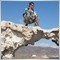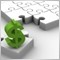Number of trades per day.1031

Is it possible to limit the number of total trades placed in a day by an EA? Also can it be possible to limit how many longs and how many shorts? Does the day for an EA start at 00:00 server time?

Thanks.7258

Is it possible to limit the number of total trades placed in a day by an EA? Also can it be possible to limit how many longs and how many shorts? Does the day for an EA start at 00:00 server time?

Thanks.

Yes. Possible.1031

How can it be done?5043

Is it possible to limit the number of total trades placed in a day by an EA? Also can it be possible to limit how many longs and how many shorts? Does the day for an EA start at 00:00 server time?

Thanks.

It is possible that and much more ... programming in MQL4 and mql51031

How do I restrict the number of trades per day? Like X number of long/short or total number.

Thanks.8796

How do I restrict the number of trades per day? Like X number of long/short or total number.

Thanks.

It has to be coded in the EA1031

Which functions do I use? Are there functions for long trades/short trades closed for day or total closed trades for day? When does an EA start the day? At 00:00 Server Time?

Thanks.